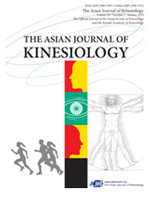상세검색
최근 검색어 전체 삭제
다국어입력
즐겨찾기0KCI등재 학술저널

# Optimal Distance of The Long Jump Approach Run Calculated Using Quadratic Regression Equation Approximation in Female College Students Attending A Physical Education Class

• 등재여부 : KCI등재
• 2019.07
• 31 - 36 (6 pages)

OBJECTIVES This study aimed to examine whether the calculation-based distance (CBD) of approach run, which was obtained using quadratic regression equation approximation, was optimal for jump distance in running long jump in female college students attending a physical education activity class. METHODS Forty-seven healthy female college students (17.2 ± 0.4 years) were included in the study. The CBDs were calculated by themselves using a graphing calculator as their learning activity. CBDs were based on four jump distances and approach-run distances (including self-selected distance [SSD]). RESULTS There was no significant difference between maximal SSD (JDSSD) and maximal CBD (JDCBD). There was no significant difference between SSD and CBD. Moreover, on average, there was no significant difference between JDSSD and JDCBD. From the viewpoint of individual differences of delta in JDCBD&#8722;JDSSD, the association with the speed-effectiveness index (SEI), as one of the jump skill metric, was investigated. The correlation coefficient between SEI and delta in JDCBD&#8722;JDSSD was not significant. CONCLUSION In this study, female college students would determine SSD as much as theoretical optimal approach-run distance (CBD) derived by their own quadratic regression model in spite of their jump skill.

Introduction

Methods

Results

Discussion

Conclusion

Conflicts of Interest

References

로딩중